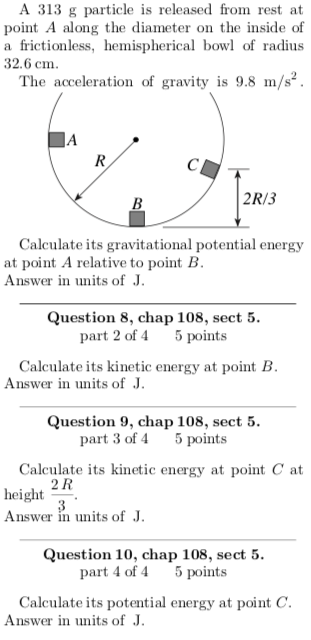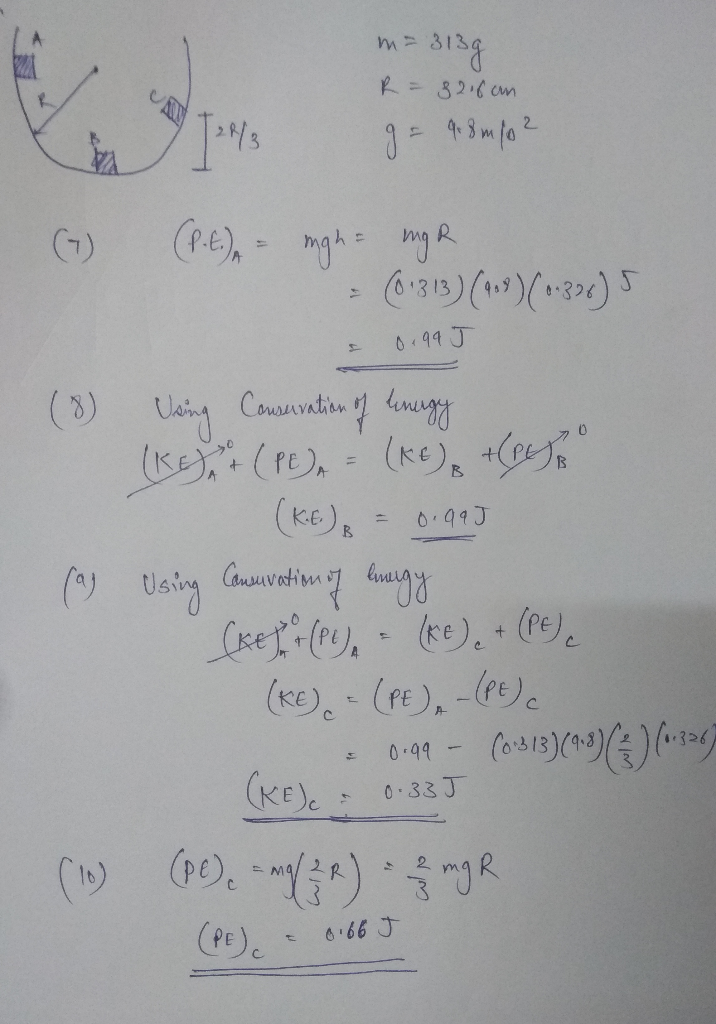Homework Help Question & Answers

# A 313 g particle is released from rest at point A along the diameter on the inside of a frictionl...A 313 g particle is released from rest at point A along the diameter on the inside of a frictionless, hemispherical bowl of radius 32.6 ст. The acceleration of gravity is 9.8 m/s 2R/3 Calculate its gravitational potential energy at point A relative to point B Answer in units ofJ Question 8, chap 108, sect 5 part 2 of 4 5 points Calculate its kinetic energy at point B Answer in units ofJ Question 9, chap 108, sect 5 part 3 of 4 5 points Calculate its kinetic energy at point C at 2 R height. Answer in units ofJ Question 10, chap 108, sect 5 part 4 of 4 5 points Calculate its potential energy at point C Answer in units ofJ##### Add Answer of: A 313 g particle is released from rest at point A along the diameter on the inside of a frictionl...
More Homework Help Questions Additional questions in this topic.

• #### (z2 + y*) dV where D is the region inside the cone z- V z2 +アbelow the plane z = 3, and inside the first ai 1- octant z 2 0,y 2 0,z2 0 (z2 + y*) dV where D is the region inside the cone z- V z2...

Need Online Homework Help?

Get FREE EXPERT Answers
WITHIN MINUTES
Related Questions